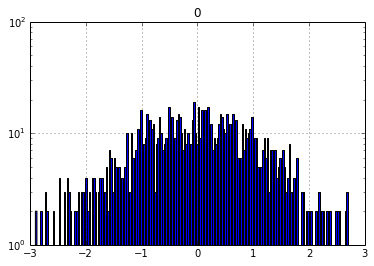-->

## Thursday, May 29, 2014

### Plotting a Logarithmic Y-Axis from a Pandas Histogram

Note to self: How to plot a histogram from Pandas that has a logarithmic y-axis. By using the "bottom" argument, you can make sure the bars actually show up. Ordinarily a "bottom" of 0 will result in no bars.
In :
import numpy as np
import matplotlib.pyplot as plt
import pandas as pd
%matplotlib inline

In :
df = pd.DataFrame(np.random.randn(1000,1))
df.hist(bins=200, bottom=1)
plt.semilogy()

Out:
[]In []:



Join my mailing list for topics on scientific computing.